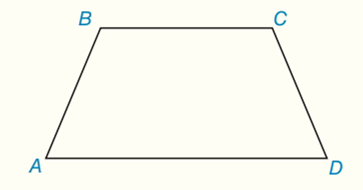Chapter 8.CR, Problem 7CRElementary Geometry For College St...

7th Edition
Alexander + 2 others
ISBN: 9781337614085

Solutions

Chapter
SectionElementary Geometry For College St...

7th Edition
Alexander + 2 others
ISBN: 9781337614085
Textbook Problem

Given: Trapezoid ABCD, with A B = 6  and  B C = 8 ,   A B ¯ ≅ C D ¯ Find: A A B C D if: a) m ∠ A = 45 ∘ b) m ∠ A = 30 ∘ c) m ∠ A = 60 ∘To determine

(a)

To find:

AABCD if mA=45

Explanation

The area A of a trapezoid whose bases have lengths b1 and b2 and whose altitude has length h is given by

A=12h(b1+b2)

Calculation:

Consider the trapezoid ABCD with AB=6 and BC=8, AB¯CD¯

Suppose mA=45

The figure is shown below:

We first find the height of the trapezoid. It is a leg of the 45-45-90 triangle containing the side of length 6, so we obtain:

h=6×22=32

We also find the lengths of AE and FD, which are equal:

AE=FD=6×22=32

The area of trapezoid is A=12h(b1+b2)

b1=8,b2=AE+EF+FD

To determine

(b)

To find:

AABCD if mA=30

To determine

(c)

To find:

AABCD if mA=60

Still sussing out bartleby?

Check out a sample textbook solution.

See a sample solution

The Solution to Your Study Problems

Bartleby provides explanations to thousands of textbook problems written by our experts, many with advanced degrees!

Get Started

Differentiate. G(y)=BAy3+B

Calculus (MindTap Course List)

In Problems 19-44, factor completely. 43.

Mathematical Applications for the Management, Life, and Social Sciences

In Exercises 29-34, rationalize the denominator of each expression. 32. a1a

Applied Calculus for the Managerial, Life, and Social Sciences: A Brief Approach

Find all possible real solutions of each equation in Exercises 3144. x31=0

Finite Mathematics and Applied Calculus (MindTap Course List)

The y-coordinate of the center of mass of the region bounded by , x = 1, x = 5, y = 0 is: 4

Study Guide for Stewart's Single Variable Calculus: Early Transcendentals, 8th

The area of the region at the right is:

Study Guide for Stewart's Multivariable Calculus, 8th